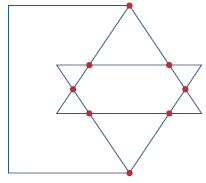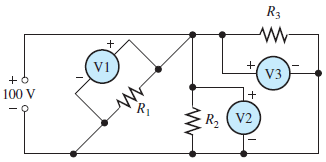# SSC JE Electrical Important MCQ Part 24

SSC JE Electrical Important MCQ PDF Part 24

1.In the network graph shown in Fig.The number of branches and nodes areA. b = 12; n = 8

B. n = 12; b = 19

C. n = 8; b = 19

D. n = 8; b = 12

(b branches, n nodes, and l independent loops

n = 12; l = 8; b = n + l –1 = 19)

2.Which of the circuits in Fig. will give Vab = 7 VA. A

B. B

C. C

D. D

Answer : D (In Circuit D Vab=+5+3-1=7 V)

3.The source voltage in Figure 61 is 100 V. How much voltage does each of the three meters readA. V1=50 V, V2=30 V, V3=20 V

B. V1=50 V, V2=20 V, V3=30 V

C. V1=50 V, V2=50 V, V3=0 V

D. V1=100 V, V2=100 V, V3=100 V

Answer : D (Voltage is the same across all parallel branches)

4.The primary method of analyzing a circuit with two or more voltage sources is usually

A. Thevenin’s theorem

B. Ohm’s law

C. Superposition

D. Kirchhoff’s law

Answer : C (Superposition theorem is a way to determine currents in a circuit with multiple sources. So superposition theorem is the primary method of analyzing a circuit with two or more voltage sources)

5.Reluctance in a magnetic circuit is analogous to

A. voltage in an electric circuit

B. current in an electric circuit

C. power in an electric circuit

D. resistance in an electric circuit

6.The unit of magnetomotive force is the

A. tesla

B. weber

C. ampere-turn

D. electron-volt

7.The merging of a free electron and a hole is called

A. Covalent bonding

C. Recombination

D. Thermal energy

8.For semiconductor material, its valence orbit is saturated when it contains

A. One electron

B. Equal (1) and (2) ions

C. Four electrons

D. Eight electrons

Answer : D (Each atom in a silicon crystal has eight electrons in its valence orbit. when the valence orbit has eight electrons, it is saturated)

9.The number of free electrons and holes in an intrinsic semiconductor decreases when the temperature

A. Decreases

B. Increases

C. Stays the same

D. None of these

Answer : A (As the temperature is decreased, electrons do not receive enough energy to break a bond and remain in the valence band. If electrons are in the conduction band they will quickly lose energy and fall back to the valence band, annihilating a hole. Therefore, lowering the temperature causes a decrease in the intrinsic carrier concentration, while raising the temperature causes an increase in intrinsic carrier concentration)

10. An acceptor atom has how many valence electrons

A.1

B. 3

C. 4

D. 5

Answer : B (An element whose each atom has 3 valence electrons is called Trivalent impurity or Acceptor impurities)

11.If you wanted to produce a p-type semiconductor, which of these would you use

A. Acceptor atoms

B. Donor atoms

C. Pentavalent impurity

D. Silicon

Answer : A (An element whose each atom has 3 valence electrons is called Trivalent impurity or Acceptor impurities. For example Indium ,Gallium,Aluminium,Boron ,etc. As they accept electrons from the covalent bonds of Si, Ge. These create p type semiconductor)

12.Which of the following describes an n-type semiconductor

A. Neutral

B. Positively charged

C. Negatively charged

D. Has many holes

Answer : A (An n-type semiconductor is formed by doping a silicon atom with a pentavalent impurity. The majority carriers in an n -type semiconductor are electrons. Atoms are always neutral in charge. In the process of doping, impurity atoms are added to a silicon atom and hence the net charge is zero)

13.When compared to a germanium diode, a silicon diode’s reverse saturation current is

A. Equal at high temperatures

B. Lower

C. Equal at lower temperatures

D. Higher

Answer : B (In germanium diodes, a small increase in temperature generates large number of minority charge carriers. The number of minority charge carriers generated in the germanium diodes is greater than the silicon diodes. Hence, the reverse saturation current in the germanium diodes is greater than the silicon diodes)

14.What causes the depletion layer

A. Doping

B. Recombination

C. Barrier potential

D. Ions

Answer : B (The combination of free electrons and holes (positive charged particles) are determined by the recombination. It is the process in which the conduction type bands free electrons jumps into the valence type band to combine with holes. So, it produces the small space of the region at junction is determined by the depletion layer. Hence, recombination process causes the depletion layer)

15.What is the barrier potential of a silicon diode at room temperature

A. 0.3 V

B. 0.7 V

C. 1 V

D. 2 mV per degree Celsius

Answer : B (At room temperature the voltage across the depletion layer for silicon is about 0.6 – 0.7 volts and for germanium is about 0.3 – 0.35 volts)

16.When comparing the energy gap of germanium and silicon atoms, a silicon atom’s energy gap is

B. Lower

C. Higher

D. Unpredictable

Answer : C (Energy bandgap, Eg  Germanium=0.74 and Silicon=1.6)

17.While maintaining a constant temperature, a silicon diode has its reverse-bias voltage increased.The diode’s saturation current will

A. Increase

B. Decrease

C. Remain the same

D. Equal its surface-leakage current

Answer : C (The saturation current of silicon depends on the junction temperature. Reverse current does not dependent the reverse voltage.The saturation current is greater when the junction temperature is higher. For every 10 ⁰C rise in temperature, the saturation current gets doubled)

18.The voltage where avalanche occurs is called the

A. Barrier potential

B. Depletion layer

C. Knee voltage

D. Breakdown voltage

Answer : D (The reverse voltage produces the avalanche effect or zener effect. Then the diode destroys due to effect of large breakdown current. Thus, the voltage occurs avalanche region in a diode is called break down voltage)

19.When the reverse voltage decreases from 10 to 5 V, the depletion layer

A. Becomes smaller

B. Becomes larger

C. Is unaffected

D. Breaks down